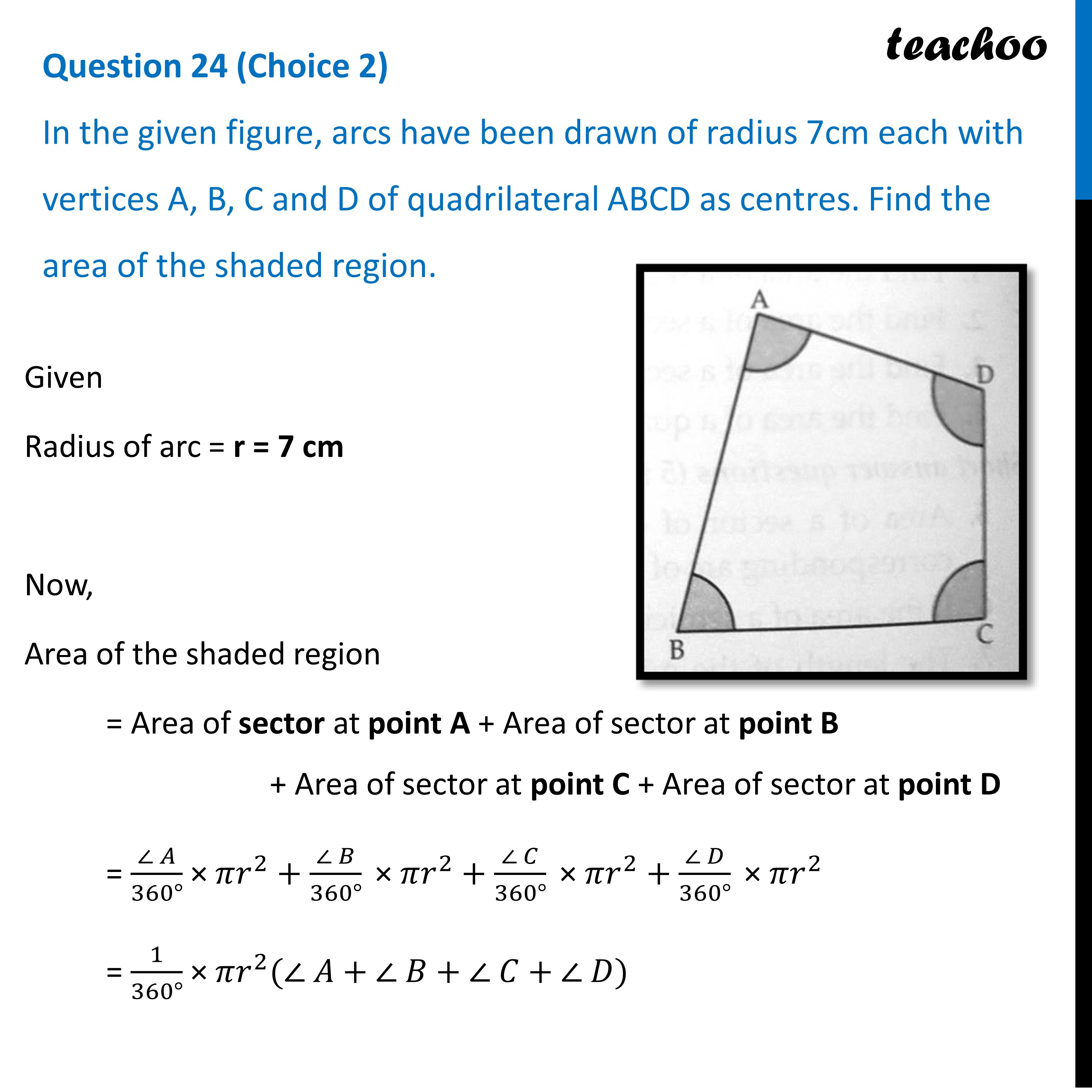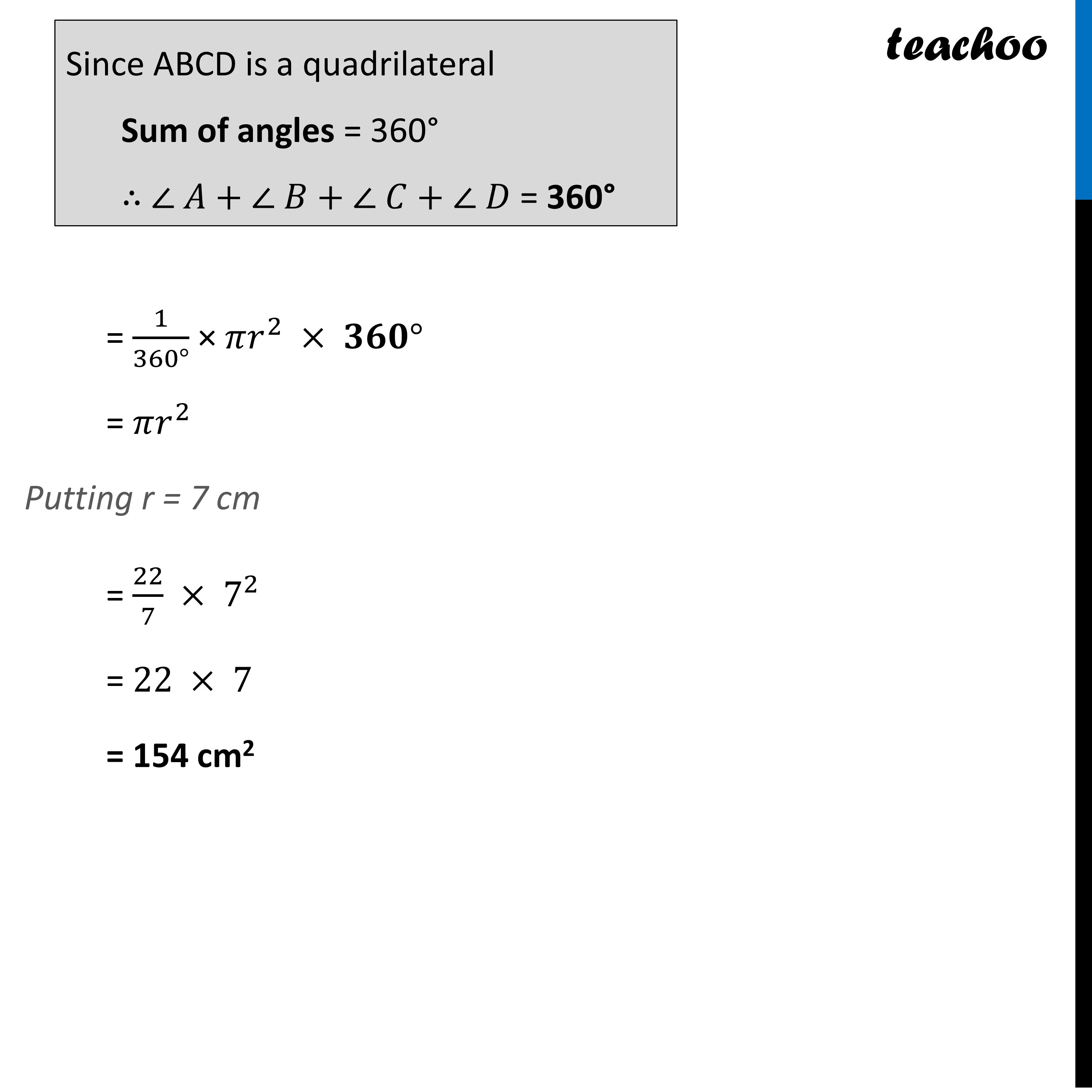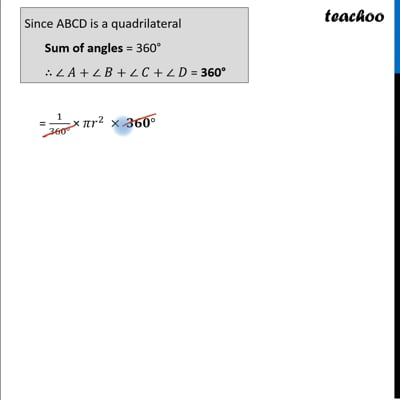CBSE Class 10 Sample Paper for 2023 Boards - Maths Standard

Class 10
Solutions of Sample Papers for Class 10 Boards

## In the given figure, arcs have been drawn of radius 7cm each with vertices A, B, C and D of quadrilateral ABCD as centres. Find the area of the shaded region.This video is only available for Teachoo black users

Get live Maths 1-on-1 Classs - Class 6 to 12

### Transcript

Question 24 (Choice 2) In the given figure, arcs have been drawn of radius 7cm each with vertices A, B, C and D of quadrilateral ABCD as centres. Find the area of the shaded region.Given Radius of arc = r = 7 cm Now, Area of the shaded region = Area of sector at point A + Area of sector at point B + Area of sector at point C + Area of sector at point D = (∠ 𝐴)/(360°) × 𝜋𝑟^2+(∠ 𝐵)/(360°) " × " 𝜋𝑟^2+(∠ 𝐶)/(360°) " × " 𝜋𝑟^2+(∠ 𝐷)/(360°) " × " 𝜋𝑟^2 = 1/(360°) × 𝜋𝑟^2 (∠ 𝐴+∠ 𝐵+∠ 𝐶+∠ 𝐷) Since ABCD is a quadrilateral Sum of angles = 360° ∴ ∠ 𝐴+∠ 𝐵+∠ 𝐶+∠ 𝐷 = 360° = 1/(360°) × 𝜋𝑟^2 × 𝟑𝟔𝟎° = 𝜋𝑟^2 Putting r = 7 cm = 22/7 × 7^2 = 22 × 7 = 154 cm2